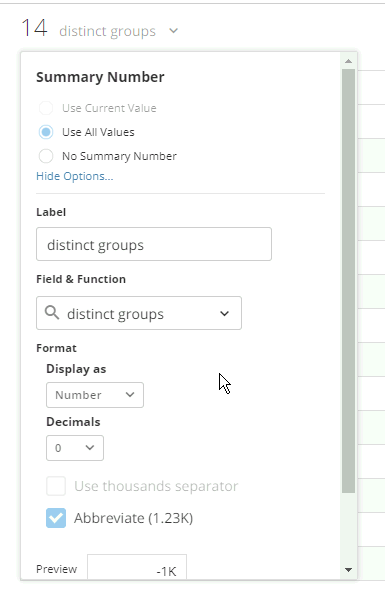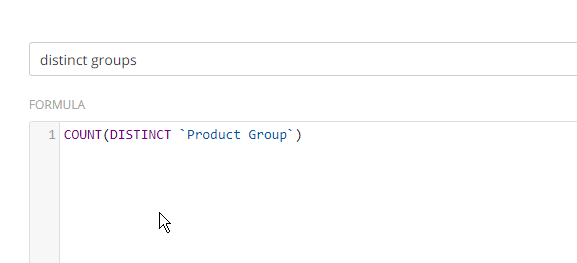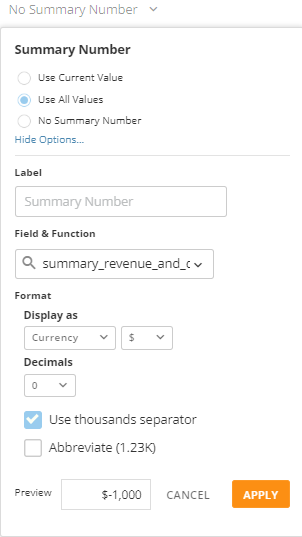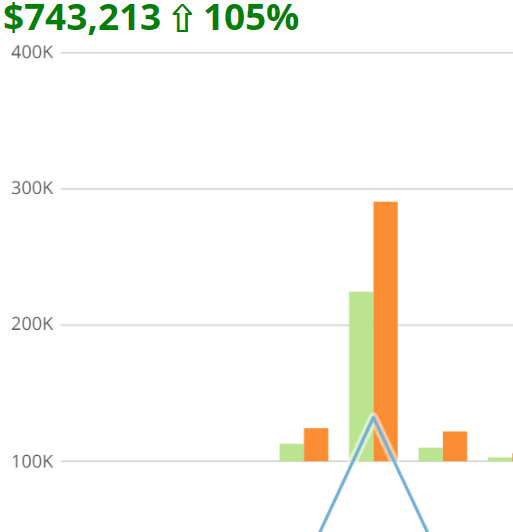# Custom Summary Number

Let me preface this by saying that I use custom summary numbers on a lot of cards and I format the html to include colors and symbols so I feel a little embarassed making this post.  Recently I have custom summary numbers that will not work on one dataset but work on another.  For the life of me I cannot figure out why, so I was hoping somebody could point out the probably obvious answer that is escaping me.

Here is a very simple example that works on one dataset but not another. The dataset that displays 'No Summary Number' with this beast mode does not have any null values for quantity so I cannot figure out why this would not work.  What else should I be looking for?  Thanks in advance for your help.

concat(case
when length(sum(`quantity`))=4 then concat(substring(sum(`quantity`),1,1),',',substring(sum(`quantity`),2,3))
else sum(`quantity`)
end)

-----------------
Chris

•CoachI'm assuming there must be more to this case statement, or else why not just use

`SUM(`quantity`)`

and format the summary number as a number with comma seperator?

That being said, could you provide a screenshot with the summary number setting for the card that isn't working?As well as the beast mode formula:“There is a superhero in all of us, we just need the courage to put on the cape.” -Superman
• Yes, this was just a basic example that should work but does not.  Below is a larger example that concats 2 measures that I want to display as the summary.  If I concat the two fields it works fine.  As soon as I put either measure inside of a case statement the summary number goes blank.

concat(
case
when length(sum(`quantity`))=7 then concat(substring(sum(`quantity`),1,1),',',substring(sum(`quantity`),2,3),',',substring(sum(`quantity`),5,3))
when length(sum(`quantity`))=6 then concat(substring(sum(`quantity`),1,3),',',substring(sum(`quantity`),4,3))
when length(sum(`quantity`))=5 then concat(substring(sum(`quantity`),1,2),',',substring(sum(`quantity`),3,3))
when length(sum(`quantity`))=4 then concat(substring(sum(`quantity`),1,1),',',substring(sum(`quantity`),2,3))
else sum(`quantity`)
end
,' tickets for \$'
,case
when sum(`net_revenue`)>999999 then concat(round(sum(`net_revenue`)/1000000,2),'m')
when sum(`net_revenue`)>999 then concat(round(sum(`net_revenue`)/1000,2),'k')
else round(sum(`net_revenue`),2)
end
)

Here is a screenshot of the summary number settings:I do have many cards wtih custom summary numbers as seen here that work so I am confused why I am having issues with such a simple beast mode.-----------------
Chris